# 1 Lecture 1

## 1.2 Intro

Prerequisites:

• Homological Algebra
• Abelian Categories
• Derived Functors
• Spectral Sequences (just exposure!)
• Sheaf theory and sheaf cohomology
• Schemes (Hartshorne II and III)

Outline/Goals:

• Basics of etale cohomology
• Etale morphism
• Grothendieck topologies
• The etale topology
• Etale cohomology and the basis theorems
• Etale cohomology of curves
• Comparison theorems to singular cohomology
• Focused on the case where coefficients are a constructible sheaf.
• Prove the Weil Conjectures (more than one proof)
• Proving the Riemann Hypothesis for varieties over finite fields

One of the greatest pieces of 20th century mathematics!

• Topics
• Weil 2 (Strengthening of RH, used in practice)
• Formality of algebraic varieties (topological features unique to varieties)
• Other things (monodromy, refer to Katz’ AWS notes)

## 1.3 What is Etale Cohomology?

Suppose $$X/{\mathbb{C}}$$ is a quasiprojective variety: a finite type separated integral $${\mathbb{C}}{\hbox{-}}$$scheme. If you take the complex points, it naturally has the structure of a complex analytic space $$X({\mathbb{C}})^{\text{an}}$$: you can give it the Euclidean topology, which is much finer than the Zariski topology. For a nice topological space, we can associate the singular cohomology $$H^i(X({\mathbb{C}})^{\text{an}}, {\mathbb{Z}})$$, which satisfies several nice properties:

• Finitely generated $${\mathbb{Z}}{\hbox{-}}$$modules
• Extra Hodge structure when tensored up to $${\mathbb{C}}$$ (same as $${\mathbb{C}}$$ coefficients)
• Cycle classes (i.e. associate to a subvariety a class in cohomology)

Goal of etale cohomology: do something similar for much more general “nice” schemes. Note that some of these properties are special to complex varieties. (E.g. finitely generated: not true for a random topological space.)

We’ll associate to $$X$$ a “nice scheme” $$\rightsquigarrow H^i(X_{\text{et}}, {\mathbb{Z}}/\ell^n{\mathbb{Z}})$$. Take the inverse limit over all $$n$$ to obtain the $$\ell{\hbox{-}}$$adic cohomology $$H^i(X_{\text{et}}, {\mathbb{Z}}_\ell)$$. You can tensor with $${\mathbb{Q}}$$ to get something with $${\mathbb{Q}}_\ell$$ coefficients. And as in singular cohomology, you can a “twisted coefficient system.”

What are some nice schemes?

• $$X = \operatorname{Spec}{\mathcal{O}}_k$$, the ring of integers over a number field.
• $$X$$ a variety over an algebraically closed field
• Typical, most analogous to taking a variety over $${\mathbb{C}}$$.
• $$X$$ a variety over a non-algebraically closed field

Some comparisons between the last two cases:

• For $${\mathbb{C}}{\hbox{-}}$$ variety, $$H^i_{\text{sing}}$$ will vanish above $$i=2d$$.
• Over a finite field, $$H^i$$ will vanish for $$i>2d+1$$ but generally not vanish for $$i=2d+1$$.

In good situations, these are finitely generated $${\mathbb{Z}}/\ell^n{\mathbb{Z}}{\hbox{-}}$$modules, have Mayer-Vietoris and excision sequences, spectral sequences, etc. Related invariants: for a scheme with a geometric1 point: $$(X, \mkern 1.5mu\overline{\mkern-1.5mux\mkern-1.5mu}\mkern 1.5mu) \rightsquigarrow \pi_1^{\text{étale}}(X, \mkern 1.5mu\overline{\mkern-1.5mux\mkern-1.5mu}\mkern 1.5mu)$$, which is a profinite topological group.

More invariants beyond the scope of this course:

• Higher homotopy groups
• Homotopy type (equivalence class of spaces)

So we want homotopy-theoretic invariants for varieties.

This cohomology theory is necessarily weird! The following theorem explains why. The slogan: there is no cohomology theory with $${\mathbb{Q}}$$ coefficients.

There does not exists a cohomology theory for schemes over $$\mkern 1.5mu\overline{\mkern-1.5mu{\mathbb{F}}\mkern-1.5mu}\mkern 1.5mu_q$$ with the following properties:

1. Functorial
2. Satisfies the Kunneth formula
3. For $$E$$ an elliptic curve, $$H^1(E) = {\mathbb{Q}}^2$$.

Take $$E$$ to be a supersingular elliptic curve. Then $$\operatorname{End}(E) \otimes{\mathbb{Q}}$$ is a quaternion algebra, and use the fact that there are no algebra morphisms $$R\to \operatorname{Mat}_{2\times 2}({\mathbb{Q}})$$.

Functoriality and Kunneth implies that $$\operatorname{End}(E)\curvearrowright E$$ yields an action on $$H^1(E)$$, which is precisely an algebra morphism $$\operatorname{End}(E) \to \operatorname{Mat}_{2\times 2}({\mathbb{Q}})$$, a contradiction.

The content here: the sum of two endomorphisms act via their sum on $$H^1$$.

Prove the same thing for $${\mathbb{Q}}_p$$ coefficients, where $$p$$ divides the characteristic of the ground field. Proof the same, just need to know what quaternion algebras show up.

This forces using some funky type of coefficients.

## 1.4 What are the Weil Conjectures?

Suppose $$X/{\mathbb{F}}_q$$ is a variety, then \begin{align*} \zeta_X(t) = \exp{\sum_{n>0} { {{\left\lvert {X({\mathbb{F}}_{q^n})} \right\rvert} \over n} t^n } } .\end{align*}

• $${\frac{\partial }{\partial t}\,} \log \zeta_X(t)$$ is an ordinary generating function for the number of rational points.

• Slogan: locations of zeros and poles of a meromorphic function control the growth rate of the coefficients of the Taylor series of the logarithmic derivative.

Make this slogan precise for rational functions, i.e. ratios of two polynomials.

1. $$\zeta_x(t)$$ is a rational function.

2. (Functional equation) For $$X$$ smooth and proper \begin{align*} \zeta_X(q^{-n} t^{-1}) = \pm q^{nE \over 2} t^E \zeta_X(t) .\end{align*}

3. (RH) All roots and poles of $$\zeta_X(t)$$ have absolute value $$q^{i\over 2}$$ with $$i\in {\mathbb{Z}}$$, and these are equal to the $$i$$th Betti numbers if $$X$$ lifts to characteristic zero.2

These are all theorems! The proofs:

1. Dwork, using $$p{\hbox{-}}$$adic methods. Proof here will follow from the fact that $$H^i_{\text{étale} }$$ are finite-dimensional. Related to the Lefschetz Trace Formula, and is how Grothendieck thought about it.

2. Grothendieck, follows from some version of Poincaré duality.

3. (and 4) Deligne.

### 1.4.1 Euler Product

Let $${\left\lvert {X} \right\rvert}$$ denote the closed points of $$X$$, then there is an Euler product: \begin{align*} \zeta_X(q^{-n} t^{-1}) = \pm q^{nE \over 2} t^E \zeta_X(t) &= \prod_{x\in {\left\lvert {X} \right\rvert}} \exp{t^{\deg (x)} + {t^{2\deg (x)} \over 2} + \cdots} \\ &= \prod_{x\in {\left\lvert {X} \right\rvert}} \exp{-\log(1-t^{\deg(x)})} \\ &= \prod_{x\in {\left\lvert {X} \right\rvert}} {1 \over 1 - t^{\deg(x)}} .\end{align*}

Check the first equality. If you have a point of $$\deg(x) = n$$, how many $${\mathbb{F}}_{q^n}$$ points does this contribute? I.e., how many maps are there $$\operatorname{Spec}({\mathbb{F}}_{q^n}) \to \operatorname{Spec}({\mathbb{F}}_{q^n})$$ over $${\mathbb{F}}_q$$?

There are exactly $$n$$: it’s $$\operatorname{Gal}({\mathbb{F}}_{q^n} / {\mathbb{F}}_q)$$. But then division by $$n$$ drops this contribution to one.

We can keep going by expanding and multiplying out the product: \begin{align*} \prod_{x\in {\left\lvert {X} \right\rvert}} {1 \over 1 - t^{\deg(x)}} &= \prod_{x\in {\left\lvert {X} \right\rvert}} (1 + t^{\deg(x)} + t^{2 \deg(x)}) \\ &= \sum_{n\geq 0} \qty{\text{\# of Galois-stable subset of $X(\mkern 1.5mu\overline{\mkern-1.5mu{\mathbb{F}}\mkern-1.5mu}\mkern 1.5mu_{q^n})$ of size $n$}}t^n .\end{align*}

Why? If you have a degree $$x$$ point, it contributes a stable subset of size $$x$$: namely the $${\mathbb{F}}_{q^n}$$ points of $${\mathbb{F}}_{q^n}$$. Taking Galois orbits like that correspond to multiplying this product. But these are the points of some algebraic variety: \begin{align*} \cdots = \sum_{n\geq 0} {\left\lvert {\operatorname{Sym}^n(X)({\mathbb{F}}_q)} \right\rvert} t^n ,\end{align*} where $$\operatorname{Sym}^n(X) \mathrel{\vcenter{:}}= X^n/\Sigma_n$$, the action of the symmetric group. Why is that? A $$\mkern 1.5mu\overline{\mkern-1.5mu{\mathbb{F}}\mkern-1.5mu}\mkern 1.5mu_q$$ point of $$\operatorname{Sym}^n(X)$$ is an unordered $$n{\hbox{-}}$$tuple of $$\mkern 1.5mu\overline{\mkern-1.5mu{\mathbb{F}}\mkern-1.5mu}\mkern 1.5mu_q$$ points without an ordering, and asking them to be Galois stable is the same as saying that they are an $${\mathbb{F}}_q$$ point.

For $$X$$ a smooth proper curve over $${\mathbb{F}}_q$$, $$\zeta_X(t)$$ is rational.

There is a set map \begin{align*} \operatorname{Sym}^n X &\to {\operatorname{Pic}}^n X \\ D &\mapsto {\mathcal{O}}(D) ,\end{align*} where here the divisor is an $$n{\hbox{-}}$$tuple of points.

What are the fibers over a line bundle $${\mathcal{O}}(D)$$? A linear system, i.e. the projectivization of global sections $${\mathbb{P}}\Gamma(X, {\mathcal{O}}(D))$$. What is the expected dimension? To compute the dimension of the space of line bundles on a curve, use Riemann-Roch: \begin{align*} \dim {\mathbb{P}}\Gamma(X, {\mathcal{O}}(D)) = \deg(D) + 1 - g + \dim H^1(X, {\mathcal{O}}(D)) - 1 .\end{align*} where the last $$-1$$ comes from the fact that this is a projective space.

If $$\deg(D) = 2g-2$$, then $$H^1(X, {\mathcal{O}}(D)) = 0$$.

This is because it’s dual to $$H^0(X, {\mathcal{O}}(K-D))^\vee$$, but this has negative degree and a line bundle of negative degree can never have sections.3 Thus the fibers are isomorphic to $${\mathbb{P}}^{n-g}$$ for $$n>2g-2$$. Now make a reduction4 and without loss of generality, assume $$X({\mathbb{F}}_q) \neq \emptyset$$. In this case, $${\operatorname{Pic}}^n(X) \cong {\operatorname{Pic}}^{n+1}(X)$$ for all $$n$$, since you can take $${\mathcal{O}}(P)$$ for $$P$$ a point, a degree 1 line bundle, and tensor with it. It’s an isomorphism because you can tensor with the dual bundle to go back. Thus for all $$n>2g-2$$, \begin{align*} {\left\lvert {\operatorname{Sym}^n(X)({\mathbb{F}}_q)} \right\rvert} = {\left\lvert {{\mathbb{P}}^{n-g}({\mathbb{F}}_q)} \right\rvert} \cdot {\left\lvert {{\operatorname{Pic}}^n(X)({\mathbb{F}}_q)} \right\rvert} = {\left\lvert {{\mathbb{P}}^{n-g}({\mathbb{F}}_q)} \right\rvert} \cdot {\left\lvert {{\operatorname{Pic}}^0(X)({\mathbb{F}}_q)} \right\rvert} .\end{align*}

Thus $$\zeta_X(t)$$ is a polynomial plus $$\sum_{n>2g-2} {\left\lvert {{\operatorname{Pic}}^n(X)({\mathbb{F}}_q)} \right\rvert}\qty{1+q+q^2+\cdots+q^{n-g}}t^n$$.

Show that this is a rational function using the formula for a geometric series.

The functional equation in the case of curves: \begin{align*} \zeta_X(q^{-1} t^{-1} ) = \pm q^{2-2g \over 2} t^{2-2g} \zeta_X(t) .\end{align*}

Where it comes from in terms of $$\operatorname{Sym}^n$$: Serre duality.

We’ll show the RH later.

Suppose $$X/{\mathbb{F}}_q$$ is any variety, then $$\zeta_X(t)$$ is rational function.

This was roughly known to Weil, hinted at in original paper

Idea: take Frobenius (intentionally vague, arithmetic vs geometric vs …) $$F:X\to X$$, then $$X({\mathbb{F}}_q)$$ are the fixed points of $$F$$ acting on $$X_{\mkern 1.5mu\overline{\mkern-1.5mu{\mathbb{F}}\mkern-1.5mu}\mkern 1.5mu_q}$$, and the $${\mathbb{F}}_{q^n}$$ points are the fixed points of $$F^n$$. Uses the Lefschetz fixed point formula, which will say for $$\ell\neq \operatorname{ch}({\mathbb{F}}_q)$$,5

\begin{align*} {\left\lvert {X({\mathbb{F}}_{q^n})} \right\rvert} = \sum_{i=0}^{2\dim(X)} (-1)^i \operatorname{Tr}(F^n) H^i_c(X_{{\mathbb{F}}_q}, {\mathbb{Q}}_\ell) .\end{align*}

\begin{align*} \exp{\sum {\operatorname{Tr}(F^n) \over n}t^n }\quad\text{is rational} .\end{align*}

This lemma implies the result, because if you plug the trace formula into the zeta function, you’ll get an alternating product $$f \cdots {1\over g} \cdot h \cdot {1\over j} \cdots$$ of functions of the form in the lemma, which is still rational.

It suffices to treat the case $$\dim(V) = 1$$, because otherwise you can just write this as a sum of powers of eigenvalues. Then you have a scalar matrix, so you obtain \begin{align*} \exp{ \sum {\alpha^n \over n} t^n} = \exp{ -\log(1 - \alpha t)} = {1 \over 1-\alpha t} ,\end{align*} which is rational.

This proves rationality, contingent on

1. The Lefschetz fixed point formula
2. The finite dimensionality of etale cohomology

Try to figure out how Poincaré duality should give the functional equation.

(Hint: try the lemma on a vector space where $$F$$ scales a bilinear form.)

Suppose $$X/{\mathbb{C}}$$ is a smooth projective variety and $$[H] \in H^2(X({\mathbb{C}}), {\mathbb{C}})$$ is a hyperplane class (corresponds to intersection of generic hyperplane or the first Chern class of an ample line bundle). Suppose $$F:X\to X$$ is an endomorphism such that $$f^*[H] = q[H]$$ for some $$q\in {\mathbb{Z}}_{\geq 1}$$.

Define \begin{align*} L(F^n) \mathrel{\vcenter{:}}= \sum_{i=0}^{2\dim(X)} (-1)^i \operatorname{Tr}\qty{ F^n {~\mathrel{\Big|}~}H^i(X_{{\mathbb{F}}_q}, {\mathbb{Q}}_\ell)} .\end{align*} and \begin{align*} \zeta_{X, F}(t) \mathrel{\vcenter{:}}= \exp{\sum_{n=1}^\infty {L(F^n) \over n}t^n } .\end{align*}

Then $$\zeta_{X, F}(t)$$ satisfies the RH: the zeros and poles are of absolute value $$q^{i\over 2}$$. Equivalently, the eigenvalues $$\lambda$$ of $$F^n$$ acting on $$H^i(X, {\mathbb{C}})$$ all satisfy $${\left\lvert {\lambda} \right\rvert} = q^{i\over 2}$$.

Next time, to review

• Étale morphisms
• Definition of a site

# 2 Lecture 2

## 2.1 Review

From last time: we want to prove the following theorem of Serre, a complex analog of the Weil conjectures. After this, we’ll talk about étale morphisms, the étale topology, and possibly the definition of étale cohomology.

Let $$X_{/{\mathbb{C}}}$$ be a smooth projective variety and $$[H]\in H^2(X; {\mathbb{Z}})$$ be a hyperplane class6 and an endomorphism $$F:X\to X$$ a map satisfying $$F^*[H] = q[H]$$ for some $$q\in {\mathbb{Z}}_{\geq 1}$$. Then the eigenvalues of $$F^*$$ on $$H^i(X;{\mathbb{C}})$$ all have absolute value $$q^{i\over 2}$$.

Note that the same $$q$$ is appearing in both parts of the theorem. Why prove this theorem? Later on, to prove the Riemann hypothesis for varieties over finite fields, we’ll prove that the Frobenius acts in this way on the étale cohomology. There is in fact a reason this is true, coming from some special properties of the behaviors of the cohomology of varieties which aren’t manifested in random topological spaces.

The proof here will not look at all like Deligne’s proof of the Riemann hypothesis for varieties over finite fields. We’ll see shadows of it, but use a lot of things that are true for complex varieties that are still not known for varieties over finite fields.

There is a cup product map \begin{align*} L: H^i(X; {\mathbb{C}}) &\to H^{i+2}(X; {\mathbb{C}}) \\ \alpha &\mapsto \alpha \smile [H] .\end{align*} Thus taking the direct sum $$\bigoplus_i H^i(X; {\mathbb{C}})$$ yields an algebra.

Each $$H^i(X; {\mathbb{C}}) \cong \operatorname{im}(L) \oplus H^i_{\text{prim}}$$, which is an isomorphism that depends on a choice of hyperplane class $$[H]$$ but is then canonically defined. Moreover, there is a Hodge decomposition $$H^i_{\text{prim}} = \bigoplus_{p+q=i}H^{p, q}_{\text{prim}}$$.

If $$\alpha, \beta \in H^k(X)_{\text{prim}}$$, then there is a natural pairing \begin{align*} {\left\langle {a},~{b} \right\rangle} = i^* \int_X a\wedge \mkern 1.5mu\overline{\mkern-1.5mu\beta\mkern-1.5mu}\mkern 1.5mu \wedge [H]^{n-k} ,\end{align*} where we’ve used the fact that the integrand is a top form and can thus be integrated. Moreover, this is a definite bilinear form on $$H^{p, q}_{\text{prim}}$$, i.e. a nonzero element paired with itself is again nonzero.

The moral of the story here is that cohomology breaks up into pieces, where $$\operatorname{im}L$$ comes from lower degrees and can perhaps be controlled inductively, and the higher dimensional pieces carry a canonical definite bilinear form.

## 2.2 Sketch proof of Serre’s analog of the Riemann hypothesis

As a reminder, we want to show that the eigenvalues of $$F^*$$ acting on $$H^k(X; {\mathbb{C}})$$ have absolute value $$q^{k\over 2}$$ where $$q$$ is the scalar associated to $$F$$ acting on $$[H]$$.

It suffices to do this for $$H^k_{\text{prim}}$$.

Why is this true? If we have an eigenvector $$\alpha\in H^{k-2}(X; {\mathbb{C}})$$, then by induction on $$k$$ we can assume the eigenvalue has absolute value $$q^{k-2 \over 2}$$. Then $$F^*(\alpha \smile [H]) = F^* \alpha \smile F^*[H] = \lambda \alpha \smile q[H] = q\lambda (\alpha \smile [H])$$, so this is an eigenvector of absolute values $$q q^{k-2\over 2} = q^{k\over 2}$$.

Now for the primitive part, let $$\alpha\in H^k_{{\operatorname{prim}}}$$ be an $$F^*$$ eigenvector. Since $$F^*$$ preserves $$H^{p, q}$$, we can assume $$\alpha \in H^{p, q}_{{\operatorname{prim}}}$$ for some $$p+q=k$$. Consider \begin{align*} {\left\langle {F^* \alpha},~{F^*\alpha} \right\rangle} .\end{align*} On one hand, this is equal to $${\left\lvert {\lambda} \right\rvert}^2 {\left\langle {\alpha},~{\alpha} \right\rangle}$$ by sesquilinearity, pulling out a $$\lambda$$ and a $$\mkern 1.5mu\overline{\mkern-1.5mu\lambda\mkern-1.5mu}\mkern 1.5mu$$. On the other hand, it is equal to \begin{align*} \cdots &= i^* \int F^* \alpha \wedge F^* \mkern 1.5mu\overline{\mkern-1.5mu\alpha \mkern-1.5mu}\mkern 1.5mu\wedge [H]^{n-k} \\ &= {i^k \over q^{n-k}} \int F^*\qty{\alpha \wedge \mkern 1.5mu\overline{\mkern-1.5mu\alpha \mkern-1.5mu}\mkern 1.5mu\wedge [H]^{n-k} } \\ &= {q^n i^k \over q^{n-k}} \int \alpha \wedge \mkern 1.5mu\overline{\mkern-1.5mu\alpha \mkern-1.5mu}\mkern 1.5mu\wedge H^{n-k} \\ &= q^k {\left\langle {\alpha},~{\alpha} \right\rangle} .\end{align*}

Using the Lefschetz hyperplane theorem or Poincaré duality, $$F^*$$ acts on $$H^{2n}(X; {\mathbb{C}})$$ via $$q^n$$.

So we’re done if $${\left\langle {\alpha},~{\alpha} \right\rangle} \neq 0$$, since this implies $${\left\lvert {\lambda} \right\rvert}^2 = q^k$$ and thus $${\left\lvert {\lambda } \right\rvert}= q^{k\over 2}$$. Why is this true? This is the statement of the Hodge index theorem.

The structures on cohomology imply this complex analog of the Riemann hypothesis, and we’ll want to use something similar for varieties over a finite field. This will be hard! Deligne doesn’t quite accomplish this: there’s no analog of the Hodge decomposition and we don’t know the Hodge index theorem.

This is the proof that will motivate much of the rest of what we’ll do in the course.

## 2.3 Étale Morphisms

This is a property of morphism of schemes, see Hartshorne.

Suppose $$f:X\to Y$$ is a morphism of schemes. Then $$f$$ is étale is it is locally of finite presentation, flat, and unramified.

$$f$$ is unramified if $$\Omega_{X/Y}^1 = 0$$ (the relative Kahler differentials). Equivalently, all residue field extensions are separable, i.e. given a point in $$Y$$ with a point in $$X$$ above it, the residue fields of these points gives a field extension, and we require it to be separable.

Suppose we have a nilpotent ideal $$I$$, so $$I^n = 0$$ for some $$n$$, then $$f:X\to Y$$ is formally étale if there is a unique lift in the following diagram:This is supposed to resemble a covering space map: We have $$\operatorname{Spec}(A) \in Y$$ with a nilpotent thickening and a map from $$A/I$$, which you may think of as a reduced subscheme. This thus says that tangent vectors downstairs can be lifted in a unique way to tangent vectors upstairs:

There are some equivalent definitions of a morphism being étale:

• Smooth of relative dimension zero

• Locally finite presentation and formally étale

• Locally standard étale, i.e. for each $$x\in X$$ with $$y\mathrel{\vcenter{:}}= f(x)$$, there exists a $$U\ni x, V\ni y$$ such that $$f(U) \subseteq V$$ and $$V=\operatorname{Spec}(R), U = \operatorname{Spec}\qty{R[x]_h / g}$$ (where we localize at $$h$$) such that the derivative $$g'$$ is a unit in $$R[x]_h$$ and $$g$$ is monic.

For this last definition, thinking of $$\operatorname{Spec}(R[x])$$ as $$R\times {\mathbb{A}}^n$$, what happens when modding out by a polynomial $$g$$? This yields a curve cutting out the roots of $$g$$. Inverting $$h$$ deletes the locus where $$h$$ vanishes, and $$g'$$ being a unit means that the $$g$$ has no double roots in the fibers. In other word, the deleted locus passes through all double roots:

Check that standard étale morphisms are étale, and try to understand the proof that all étale morphisms are locally standard étale.

• Multiplication by $$[n]$$ on an elliptic curve if $$n \in {\mathbb{Z}}$$ is invertiable in the base field.

• Take $${\mathbb{G}}_m = \operatorname{Spec}k[t, t^{-1}]$$, and the map \begin{align*} {\mathbb{G}}_m &\to {\mathbb{G}}_m \\ t^m &\mapsfrom t ,\end{align*} where $$n$$ is prime to $$\operatorname{ch}(k)$$.7

Show that the last map above is étale.

(Hint: use the fact that $${\frac{\partial }{\partial t}\,} (t^n) = nt^{n-1}$$, which is a unit.)

Consider the map \begin{align*} {\mathbb{G}}_m &\hookrightarrow{\mathbb{A}}^1 \\ k[t, t^{-1}] &\mapsfrom k[t] .\end{align*} We need to check 3 things:

• Locally finite presentation,
• This is a finitely presented ring map, since you just need to adjoin an inverse of $$t$$, one element and one relation.
• Flat,
• Since open embeddings are flat,
• $$\Omega^1_{{\mathbb{G}}_m / {\mathbb{A}}^1} = 0$$,
• True for a Zariski open embedding.

Note that this is finite onto its image.

Any open immersion is étale.

Note that we actually already checked this!

Use the fact that $${\mathbb{G}}_m$$ is $${\mathbb{A}}^1\setminus\left\{{\mathbf{0}}\right\}$$, so take $${\mathbb{G}}_m \setminus\left\{{1}\right\}$$ and the map \begin{align*} {\mathbb{G}}_m\setminus\left\{{1}\right\} &\to {\mathbb{G}}_m \\ t^2 &\mapsfrom t .\end{align*}

What’s the picture? For the squaring map, there are two square roots:

This is an étale surjection but not finite étale, since it is not proper. This also gives a counterexample to étale morphisms always looking like covering spaces, since here that may be true locally but doesn’t hold globally.

This is an important example to keep in mind, because you’ll often see arguments that treat étale maps as though they were finite onto their image.

Take a finite separable field extension, taking $$\operatorname{Spec}$$ of it yields an étale map.

Now for some non-examples:

Take $$X = \operatorname{Spec}k[x, y] / xy$$, which looks like the following: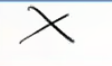$$X$$

Then the normalization $$\tilde X\to X$$ is not étale, since it is not flat.

Take the map \begin{align*} {\mathbb{A}}^1 &\to {\mathbb{A}}^1 \\ t^2 &\mapsfrom t .\end{align*}

The picture is the following:

This is note étale since it is ramified at zero. We can compute \begin{align*} \Omega_f^1 = k[t]\, dt / d(t^2) = k[t] dt/ 2t\, dt ,\end{align*} where $$2t\,dt$$ does not generate this module. This is supported at $$t=0$$ if $$\operatorname{ch}\neq 2$$.

A finite flat morphism such that $$\Omega_{X/Y}^1$$ is not torsion: a hint is that the previous example almost works, with a slight modification. Let $$\operatorname{ch}k = p$$, and take \begin{align*} {\mathbb{A}}^1 &\xrightarrow{F} {\mathbb{A}}^1 \\ t^p &\mapsfrom t .\end{align*} Then $$\Omega_f^1 = k[t]\, dt / d(t^p) = k[t]\,dt$$ since $$d(t^p) = 0$$ in characteristic $$p$$. This yields line bundles (?), so it is not torsion.

This map has a name: the relative Frobenius. In general, looking at Frobenii, the Kahler differentials will be very big. You might not be used to this: in characteristic zero, a map of relative dimension zero is generically étale. In this case, the Kahler differentials will always be torsion.

Consider a map \begin{align*} {\mathbb{A}}^m &\xrightarrow{f_1, \cdots, f_m} {\mathbb{A}}^m ,\end{align*} since such a map is given by a system of $$m$$ polynomials in $$m$$ variables. Then $$f$$ is étale is a neighborhood of $$\mathbf{a}$$ if $$\det \qty{{\frac{\partial f_i}{\partial x_j}\,} \Big|_{\mathbf{a}} }$$ is a unit.

### 2.3.1 Properties of Étale Morphisms

1. Open immersions are étale
2. Compositions of étale morphisms are étale8
3. Base change of étale morphisms are étale, i.e.1. There is a 2 out of 3 property: If $$\phi \circ \psi$$ and $$\phi$$ are étale, then $$\psi$$ is étale.

Show property 4 above.

Étale morphisms on varieties over an algebraically closed field induce isomorphisms on complete local rings at closed points.

Prove this! Hint: use the criterion for formal étaleness. There’s an evident map one way on local rings coming from your étale morphism, and you need to produce the inverse map.

$$\danger$$ If $$\psi$$ is étale and $$\phi\circ\psi$$ is étale, it is not necessarily the case that $$\phi$$ is étale. Come up with an example!

Any property that can be checked at the level of complete local rings is true for the source of an étale morphism if it is true for the target.

Why? If you want to check a property for complete local rings on the source, note that the map induces an isomorphism of complete local rings.

## 2.4 Generalizing Topologies

### 2.4.1 Sites

The notion of a site will be a generalization of topological spaces and sheaves. The idea is we’ll generalize sheaf cohomology to this setting. On a nice space like a manifold, singular cohomology is isomorphic to the sheaf cohomology of the constant sheaf $$\underline{{\mathbb{Z}}}$$. Here we’ll find some version of a sheaf where étale cohomology with $${\mathbb{Z}}/\ell^n{\mathbb{Z}}$$ coefficients will be the sheaf cohomology with the constant sheaf $$\underline{{\mathbb{Z}}/\ell^n{\mathbb{Z}}}$$.

What parts of the definition of a topological space are needed to define the notion of a sheaf?

Recall that the sheaf condition is given in two parts:

1. A section is determined by its value on a cover, and

2. Sections can be glued when they agree on intersections.

1. As in presheaves, a notion of open sets and inclusions. (I.e., a category of open sets.)910

We’d also like to make sense of the sheaf condition:

1. Collections of morphisms which are “covers,” remembering which collections of opens cover a space, and

2. The existence of certain fiber products (intersections).

The motivation for (3) above is that for $$U, V \subseteq X$$, we can form $$U\times V = U\cap V$$.

We will make the following preliminary definition:

A category $$\mathcal{C}$$ with a collection of covering families11 \begin{align*} \left\{{X_\alpha \xrightarrow{f_\alpha} X {~\mathrel{\Big|}~}\alpha\in A}\right\} \end{align*} such that several axioms are satisfied.

We’ll discuss the axioms next time, they just capture the notion of what a cover of a topological space should look like.

There are at least three different notions of this definition! The one above is perhaps the least general but the easiest to work with.

For $$X$$ a topological space, $$\mathcal{C}$$ the category of open sets in $$X$$, then $$\left\{{U_\alpha\to U}\right\}$$ is a covering family if the $$U_\alpha$$ cover $$U$$, i.e. $$U = \cup_\alpha U_\alpha$$.

Let $$M$$ be a manifold and $$\mathcal{C}$$ be the category of manifolds over $$M$$, so all $$M' \xrightarrow{f} M$$ such that $$f$$ is locally an isomorphism. Note that these are smooth local homeomorphisms. Let \begin{align*} \left\{{M_\alpha \xrightarrow{f_\alpha} M}\right\} \text{ if } \bigcup_\alpha \operatorname{im}(f_\alpha) = M \end{align*}

Let $$X$$ be a scheme and consider $$X_{\text{et}}$$ the category of all étale $$Y/X$$: so the objects are schemes $$Y$$ admitting an étale morphism $$Y\to X$$. Then $$\left\{{X_\alpha\to X}\right\}$$ is a covering family if $$\cup\operatorname{im}(f_\alpha) = X$$.

This will be the fundamental object, and we’ll define étale cohomology by defining sheaves on this category, taking a constant sheaf $$\underline{{\mathbb{Z}}/\ell^n{\mathbb{Z}}}$$, and we’ll take sheaf cohomology.

# 3 Lecture 3

## 3.1 Defining Sites

Today: we’ll discuss sites, which generalizes the category of open sets over a topological space. The goal is to generalize spaces and sheaves to categories, and to define a sheaf we need

1. A notion of a cover, and

2. A notion of intersections/fiber products of open sets.

A Grothendieck topology on $$\mathcal{C}$$ or a site on $$\mathcal{C}$$ is the data of for each $$X\in \mathrm{Ob}(\mathcal{C})$$ a collection of sets of morphism $$\left\{{X_\alpha \to X}\right\}$$ (covering families) satisfying

• Intersections exist: If $$X_\alpha\to X$$ appears in a covering family and $$Y\to X$$ is arbitrary, the fiber product $$X_\alpha\times_X Y$$ exists.

• Intersecting with a cover again yields a cover: If $$\left\{{X_\alpha\to X}\right\}$$ is a covering family and $$Y\to X$$ is arbitrary, then the covering family can be pulled back: $$\left\{{Y\times_X X_\alpha\to Y}\right\}$$ is again a covering family.12

• Compositions of coverings are again coverings: If $$\left\{{X_\alpha\to X}\right\}_{\alpha}$$ and $$\left\{{X_{\alpha\beta} \to X_\alpha}\right\}_{\alpha,\beta}$$ are covering families, then you can compose, i.e. taking the set of all possible ways of composing $$\left\{{X_{\alpha\beta} \to X_\alpha \to X}\right\}$$ is again a covering family.13

• Isomorphisms are covers: If $$X\xrightarrow{\sim_f} Y$$ is an isomorphism, then the singleton family $$\left\{{X\xrightarrow{f} Y}\right\}$$ is a covering family.

### 3.1.1 Examples of Sites

If $$X$$ is a topological space, define $$\mathcal{C}$$ whose objects are open subsets of $$X$$ where there is a unique morphism $$U\to V$$ iff $$U\subseteq V$$. Then $$\left\{{U_\alpha \to U}\right\}$$ is a covering family if $$\bigcup_\alpha U_\alpha = U$$.

Let $$X$$ be a scheme, and define the small étale site $$X_{\text{ét}}$$: the category whose objects are étale morphisms $$Y\xrightarrow{f} X$$ where morphisms are maps over $$X$$:Note that $$g$$ is étale by the 2 out of 3 property.

Then $$\left\{{X_\alpha\to X}\right\}$$ is a covering family if the set theoretic images satisfy $$\bigcup_\alpha \operatorname{im}(f_\alpha) = X$$.

Again let $$X$$ be a scheme, and define $$X_{\mathrm{Et}}$$ the category whose objects are all $$X{\hbox{-}}$$schemes (where we no longer require the maps to be étale). In other words, this is the overcategory of $$X$$: the category of schemes over $$X$$. Then $$\left\{{U_\alpha\xrightarrow{f_\alpha}U}\right\}$$ is a covering family if all of the $$f_\alpha$$ are étale and $$\bigcup_\alpha \operatorname{im}(f_\alpha) = U$$.

Note the difference: in the small site, we included only étale $$X{\hbox{-}}$$schemes, vs all $$X{\hbox{-}}$$schemes in the big site. In both cases, the notion of covering families are the same.

Let $$X$$ be a complex analytic space (e.g. a complex manifold), then there is an analytic étale site whose objects are complex analytic spaces $$Y\xrightarrow{f} X$$ such that locally on $$Y$$, $$f$$ is an analytic isomorphism. Note that this includes covering spaces. The morphisms will be morphisms over $$X$$ creating commuting triangles, and the covers are the usual covers.

This category is part of what motivates the definition of the étale topology. This is what we’re trying to imitate. E.g. if you have a complex algebraic variety, taking its analytification will be one of these. This site will show up later when we compare étale cohomology to singular cohomology.

We haven’t said what it means to be a sheaf yet, but Grothendieck topologies behave in somewhat unexpected ways. The category of sheaves of the analytic étale cohomology, $${\operatorname{Sh}}(X_{\mathrm{an{\hbox{-}}et}})$$, is canonically equivalent to $${\operatorname{Sh}}(X^{\mathrm{top}})$$. Thus sometimes the category of sheaves over a site doesn’t remember the site, i.e. two different sites can have the same category of sheaves. On the RHS we had a category of open subsets, whereas on the LHS we included things like covering spaces. We’ll see later that there is a notion of morphisms of sites, and there is a morphism inducing this equivalence.

Proving this isomorphism will be an exercise, here’s an outline of why it’s true: suppose you have a cover of $$X$$ in this category, i.e. a family of local analytic isomorphisms. Given any of these, you can cover by subsets for which these are isomorphisms onto their images.

The letter fppf stand for faithfully flat and finite presentation.14

There are small and big sites here: we define $$X_{\mathrm{\operatorname{fppf}}}$$ whose objects are fppf morphism $$Y\to X$$, with morphisms as triangular diagrams of morphisms over $$X$$, and covers are the usual covers. Note that replacing fppf morphisms with flat morphisms would yield an equivalent definition here.

If $$X$$ is a scheme, then the small Zariski topology is $$X_{\mathrm{zar}}$$ whose objects are $${\operatorname{Op}}(X^{\mathrm{top}})$$, the Grothendieck topology of the corresponding topological space, and we take the usual notion of covers. There is a big Zariski topology $$X_{\mathrm{Zar}}$$ whose category is all $$X{\hbox{-}}$$schemes $$\left\{{U_\alpha\xrightarrow{f_\alpha} U}\right\}$$ with $$f_\alpha$$ open embeddings and $$\bigcup_\alpha \operatorname{im}(f_\alpha) = U$$.

Some other examples:

• The Nisnevich topology, which lives between the Zariski and the étale topology,

• The crystalline site, used to define crystalline cohomology,

• The infinitesimal site,

• The cdh topology, the arc topology, the rh topology, and many more.

## 3.2 Toward Sheaves of Sites

For $$\mathcal{D}$$ a category, a $$\mathcal{D}{\hbox{-}}$$valued presheaf is a contravariant function $$F:\mathcal{C}\to \mathcal{D}$$.

This makes no reference to any Grothendieck topology.

If $$X$$ is a topological space, a $$\mathcal{D}{\hbox{-}}$$valued presheaf of $$X$$ is equivalent to a presheaf on $${\operatorname{Op}}(X)$$.

We can now define a sheaf. What’s the motivation? For $$X$$ a topological space, it’s a sheaf satisfying some conditions: its sections are determined by an open cover, and given sections agreeing on overlaps allows gluing. This can be captured by a specific diagram, which is what we will use here.

Recall the definition of a site (): in short, a category equipped with the Grothendieck topology.

A sheaf $$F$$ is presheaf such that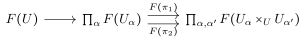is an equalizer diagram for all covering families $$\left\{{U_\alpha \to U}\right\}$$.

The diagram arises due to the fact that if we have a bunch of maps coming from a cover, since we have a contravariant functor, we get a map into the product. We then look at the intersections of all $$U_{\alpha}, U_{\alpha'}$$.

The two arrows occurring come from the projections:where we use the fact that since $$F$$ is a contravariant functor, it induces maps going the other way.

What does being an equalizer mean, say if $$F$$ is set-valued? “Exactness” at the middle term is the gluing condition, and exactness at the first term is injectivity, i.e. a section (the values of $$F$$ on $$U$$) are determined by its values on a cover (by $$F(U_\alpha)$$). Note that in fact $$F(U)$$ is the limit of this diagram. The gluing condition is more precisely that if we’re given $$(s_\alpha) \in \prod_\alpha F(U_\alpha)$$ such that $$F(\pi_1)(s_\alpha) = F(\pi_2)(s_\alpha)$$, then $$(s_\alpha)$$ comes from $$F(U)$$.

A morphism $$F_1\to F_2$$ of either presheaves or sheaves is a natural transformation of functors.

### 3.2.1 Examples of Sheaves of Sites

Any representable functor is a sheaf on the étale site $$X_{\text{Ét}}$$. In fact, any such functor is a sheaf on the big fppf site $$X_{\mathrm{\operatorname{Fppf}}}$$: the category of all $$X{\hbox{-}}$$schemes with covers as fppf covers, which are maps that are flat and jointly surjective.

Take $$\mu_n$$ the functor represented by $$\mu_n \mathrel{\vcenter{:}}=\operatorname{Spec}k[t] / t^{n-1}$$. For $$U$$ an $$X{\hbox{-}}$$scheme, we can evaluate in the following way: \begin{align*} \mu_n(U) = \left\{{f\in {\mathcal{O}}_U(U) {~\mathrel{\Big|}~}f^n = 1}\right\} .\end{align*}

We define a sheaf of the étale site as $${\mathcal{O}}^{\text{ét}}_X(U) = {\mathcal{O}}_U(U)$$ where we’ve said what the values are. This is a sheaf that is represented by $${\mathbb{A}}^1_{/X}$$.

The constant sheaf $$\underline{\mathbb{Z}/\ell^n\mathbb{Z}}$$. How can we prove it is a sheaf, given the theorem, and determine what its values are? This is represented by $$\qty{\mathbb{Z}/\ell^n\mathbb{Z}} \times X$$, i.e. taking the disjoint union of $$\ell^n$$ copies of $$X$$. The values are given by \begin{align*} \underline{\mathbb{Z}/\ell^n\mathbb{Z}}(U) = \hom_{{\operatorname{Top}}}(U^{{\operatorname{Top}}}, \mathbb{Z}/\ell^n\mathbb{Z}) ,\end{align*} where we give the set $$\mathbb{Z}/\ell^n\mathbb{Z}$$ the discrete topology and take morphisms to be continuous maps.

The constant sheaf $$\underline{S}$$ doesn’t associate $$S$$ to every open set: it instead associates $$S^d$$ where $$d$$ is the number of components. The former would only be a presheaf, and not a sheaf.

We can take the sheaf $${\mathbb{G}}_m(U) \mathrel{\vcenter{:}}={\mathcal{O}}_U(U)^{\times}$$, whose values are obtained by taking the global sections of the structure sheaf and only keeping the units. This is represented by \begin{align*} {\mathbb{G}}_{m, X} = \operatorname{Spec}{\mathbb{Z}}[t, t^{-1}] \underset{\scriptscriptstyle {\operatorname{Spec}{\mathbb{Z}}} }{\times} X \end{align*} Why does this represent this functor? Mapping into this requires that $$t$$ goes to an invertible function, which yields the isomorphism.

Note that all of these functors take values in abelian groups, which is a consequence of the fact that the representing objects are group schemes. In fact, one definition of a group scheme is that the functor it represents factors through groups.

Take the functor $${\mathbb{P}}^n(U) \mathrel{\vcenter{:}}=\hom(U, {\mathbb{P}}^n)$$. This functor can be written down as a line bundle on $$U$$ with a surjective map from $${\mathcal{O}}_U \to {\mathcal{O}}_U^n$$ (?), the functor represented by projective space, and that’s also a sheaf that is necessarily representable but not an abelian one.

Some things we still need to get to:

• A proof that $$\underline{\mathbb{Z}/\ell^n\mathbb{Z}}$$ is actually a sheaf,
• A proof that the category of sheaves on the big étale site $$X_\text{Ét}$$15 with values in $$\mathbf{Ab}$$ is abelian and has enough injectives.

## 3.3 Étale Cohomology: A Preliminary Definition

Let $$\mathcal{F}$$ be a sheaf and define a functor $$\Gamma_X: \mathcal{F}\to \mathcal{F}(X)$$ sending it to its values on $$X$$. Then the étale cohomology of $$X$$ is defined by \begin{align*} H^i(X_\text{ét}, \underline{\mathbb{Z}/\ell^n\mathbb{Z}}) \mathrel{\vcenter{:}}= R^i \Gamma_X(\underline{\mathbb{Z}/\ell^n\mathbb{Z}}) ,\end{align*} the right-derived functors of $$\Gamma_X$$.

This definition is incomplete, and in particular, it’s highly non-obvious that this category of abelian sheaves is abelian. E.g. usually when proving that the category of abelian sheaves on a topological space has cokernels, you use sheafification: you take the cokernel of a map of presheaves, which is a presheaf, and sheafify it. Here, we don’t know how to sheafify a presheaf on a site. The usual construction involves forming the espace étalé and taking sections does not work for a site, you need a genuinely different argument.

Even showing cokernels exist in the category of abelian sheaves on a site is nontrivial. Try as an exercise!

What will be true in general is that this category will be an $$AB5$$ abelian category, and having enough injectives is all that’s additionally needed. This comes from machinery developed in Grothendieck’s Tohoku paper, and we’ll sketch part of the proof.

Properties of these sheaves are not so obvious, and depend on the site you’re working over:

Consider the map \begin{align*} {\mathbb{G}}_m &\to {\mathbb{G}}_m \\ t^m &\mapsfrom t ,\end{align*} where $$n$$ is invertible over the base, e.g. if we’re over a field of characteristic coprime to $$n$$. This yields a map of sheaves in two different settings. In $$X_{\mathrm{Zar}}$$ we have \begin{align*} {\mathcal{O}}^{\times}&\to {\mathcal{O}}^{\times}\\ f &\mapsto f^n .\end{align*}

We can look at this in $$X_{\text{ét}}$$, yielding \begin{align*} {\mathcal{O}}_\text{ét}^{\times}&\to {\mathcal{O}}_\text{ét}^{\times}\\ f &\mapsto f^m .\end{align*}

This map is not an epimorphism on $$X_{\mathrm{Zar}}$$ but is on $$X_{\text{ét}}$$

It suffices to give one example: take $$X = \operatorname{Spec}{\mathbb{R}}$$ and $$n=2$$, and since this is just a point, the sheaf is determined by its values. So is the map \begin{align*} {\mathbb{R}}^{\times}&\to {\mathbb{R}}^{\times}\\ t &\mapsto t^2 \end{align*} surjective? The answer is no, of course, since its image is $${\mathbb{R}}_{\geq 0}$$.

This will be surjective on $$X_{\text{ét}}$$ if $$n$$ is invertible on $$X$$. If we were in usual topological spaces, we would want to show that given any section of the sheaf on an open set, it can be refined. Here we want to pass to an étale cover so that section has an $$n$$th root. So given $$f\in {\mathbb{G}}_m(U)$$, we want an étale cover of $$U$$ so that $$f$$ obtains an $$n$$th root. An invertible function is a map $$U\to {\mathbb{G}}_m$$, and we can form the squareThe RHS map is étale since $$n$$ is invertible, and the fiber product is étale since étale morphisms are preserved by base change.

$$f$$ has an $$n$$th root upstairs. (Verify!)

There’s a more concrete way of writing this: note that $${\mathbb{A}}^1_{U, z} = \operatorname{Spec}k[t]$$, so take the subscheme cut out by $$V(z^n - f)$$. This will be an étale cover of $$U$$ (the same one in fact) and $$z$$ is now an $$n$$th root.

Check the details! Namely that this argument implies that this map of sheaves is an epimorphism.

This map of sheaves $${\mathbb{G}}_m \xrightarrow{z^m \mapsfrom z} {\mathbb{G}}_m$$, noting that if $$n$$ is not invertible this will not be an epimorphism, will always be an epimorphism in $${\operatorname{Sh}}(X_\mathrm{\operatorname{fppf}})$$ since this map is flat and finitely presented.

## 3.4 Preview: Morphisms of Sites

Suppose $$T_1, T_2$$ are sites (categories with covering families), then a continuous map of sites $$f:T_1 \to T_2$$ is a functor $$T_2 \to T_1$$16 that preserves fiber products and sends covering families to covering families.

A continuous map $$f\in {\operatorname{Hom}}_{\operatorname{Top}}(X, Y)$$ induces a map \begin{align*} {\operatorname{Op}}(Y) &\to {\operatorname{Op}}(X) \\ U &\mapsto f^{-1}(U) .\end{align*}

Check that this is a continuous map of sites.

Next time: a bunch of examples.

# 4 Lecture 4: Descent

Last time: sites, sheaves, and morphisms of sites. Today: descent, which is how we’ll see that many familiar objects are sheaves on the étale site, such as representable functors or quasicoherent sheaves.

## 4.1 Reminder

Given two sites $$(C, T_1)$$ and $$(D, T_2)$$ where $$C, D$$ are categories and the $$T_i$$ are collections of covering families, a morphism is a functor $$f^{-1} :D\to C$$ such that

1. $$f^{-1}$$ preserves fiber products.
2. $$f^{-1}$$ sends covering families to covering families.

The main example: for $$f:X\to Y$$ a map of topological spaces, the functor $$F: {\operatorname{Op}}(Y) \to {\operatorname{Op}}(X)$$ given by $$F(U) \mathrel{\vcenter{:}}= f^{-1}(U)$$ sending an open set to its preimage.

Check that this is a continuous map of sites.

Suppose $$X$$ is a scheme, then there is a natural map of sites from $$X_{\mathrm{\operatorname{Fppf}}}$$ (all $$X{\hbox{-}}$$schemes where covers are collections of jointly surjective flat morphism) to $$X_{\text{Ét}}$$ (all $$X{\hbox{-}}$$schemes where covers are jointly surjective étale morphisms) to $$X_{\text{ét}}$$ (étale $$X{\hbox{-}}$$schemes with morphisms over $$X$$) to $$X_{{\mathrm{zar}}}$$ (the open subsets of $$X$$ with morphisms given by inclusions and covers the usual covers). The maps here are inclusions going the other way.

Check that these are continuous maps of sites.

On terminology:

• What we’ve been calling a site or a Grothendieck topology is sometimes called a Grothendieck pretopology. The major difference is that you don’t have to assume the existence of fiber products.

• You may also see the notion of a topos, which is the category of sheaves on a site.

## 4.2 Setting up Descent

1. We’ve said what it means to be a sheaf on a site, how do we check that a given functor is a sheaf on $$X_{\text{ét}}$$ or $$X_{\mathrm{\operatorname{fppf}}}$$?

2. How do we construct such sheaves?

1. If $$Y$$ is an $$X{\hbox{-}}$$scheme (i.e. a scheme equipped with a map to $$X$$) then the functor $$Z \to \hom_X(Z, Y)$$ is a sheaf on $$X_{\mathrm{\operatorname{Fppf}}}, X_{\text{Ét}}, X_{\text{ét}}$$, etc.

2. Suppose $$\mathcal{F}$$ is a quasicoherent sheaf on $$X$$, so $$\mathcal{F}\in {\mathrm{QCoh}}(X)$$, the functor of taking global sections:is a sheaf on $$X_{\mathrm{\operatorname{Fppf}}}, X_{\text{Ét}}, X_{\text{ét}}$$, etc.

The sheaf associated to the above functor on $$X_{\text{ét}}$$ will be denoted $$\mathcal{F}^\text{étale}$$.

Suppose we have an fppf cover of $$X$$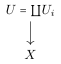Suppose $$\mathfrak{F}\in {\mathrm{QCoh}}(X)$$. When does it come from a quasicoherent sheaf on $$X$$? I.e., when is there a quasicoherent sheaf $$\mathcal{F}'$$ on $$X$$ and an isomorphism between its pullback to $$U$$ and $$\mathcal{F}$$? What extra structure do you need to “descend” to $${\mathrm{QCoh}}(X)$$, i.e. to pick such an isomorphism?

Given $$\mathcal{F}_1, \mathcal{F}_2 \in {\mathrm{QCoh}}(U)$$ and a morphism $$f: { \left.{{\mathcal{F}_1}} \right|_{{U}} } \to { \left.{{\mathcal{F}_2}} \right|_{{U}} }$$, when does $$f$$ come from $$X$$?

If $$X = \operatorname{Spec}k$$ and $$U$$ is a finite separable extension, then this question is exactly what Galois descent is about!

$$U = {\coprod}U_i \to X$$ is a Zariski cover. If we have a sheaf on $$U$$, what extra data do we need to get a sheaf on $$X$$? We need isomorphisms $${ \left.{{\mathcal{F}_i }} \right|_{{U_i\cap U_j}} } \xrightarrow{\sim} { \left.{{\mathcal{F}_j}} \right|_{{U_i\cap U_j}} }$$ (gluing data) where each $$\mathfrak{F}_i$$ is the sheaf given on $$U_i$$. We also need a cocycle condition on triple intersections. Given this data, gluing yields a sheaf on $$X$$. This may be familiar from vector bundles. Thus to give a sheaf, it suffices to specify gluing data.

Morphisms $$\mathcal{F}\to \mathcal{G}$$ is the same as morphisms $${ \left.{{\mathcal{F}}} \right|_{{U_i}} } \to { \left.{{\mathcal{G}}} \right|_{{U_i}} }$$ which commute with the gluing data. We’d like to generalize this notion of commuting with gluing data to more general types of covers.

Suppose $$U\xrightarrow{f}X$$ is a morphisms, then descent data for a quasicoherent sheaf on $$U/X$$ is the following:

1. A sheaf $$\mathcal{F}\in {\mathrm{QCoh}}(U)$$.
2. Gluing data: If we take the fiber product of $$U$$ with itself, mapping to $$U$$ under 2 different projections17,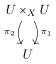there are isomorphisms \begin{align*} \phi: \pi_1^* \mathcal{F} \xrightarrow{\sim} \pi_2^* \mathcal{F} .\end{align*}

1. Cocycle condition: in the following fiber productwe have $$\pi_{23}^* \phi \circ \pi_{12}^* \phi = \pi_{13}\phi$$.

Check that this agrees with the previous notions when $$U\to X$$ is a Zariski cover.

Given descent data $$(\mathcal{F}, \phi)$$ and $$(\mathcal{G}, \psi)$$, a morphism is a morphism $$h: \mathcal{F} \to \mathcal{G}$$ of quasicoherent sheaves on $$U$$ such that the following diagram commutes:## 4.3 Descent Data is Equivalent to Quasicoherent Sheaves

Suppose $$f: U\to X$$ is fppf. Then $$f^*$$ induced an equivalence of categories between $${\mathrm{QCoh}}(X)$$ and descent data on $$U/X$$.

This doesn’t quite make sense, since we haven’t covered how to get descent data from a given sheaf. Explicitly, given $$\mathcal{F}\in {\mathrm{QCoh}}(X)$$, we can pullback to obtain $$f^* \mathcal{F}\in {\mathrm{QCoh}}(U)$$. We now want an isomorphism \begin{align*} \qty{f\circ \pi_1}^* \mathcal{F} \xrightarrow{\sim} \qty{f\circ \pi_2}^* \mathcal{F} \end{align*} on $$U\times_X U$$. We have a situation like the following: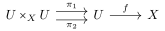Since $$f\circ \pi_1 = f\circ \pi_2$$ in this case, pulling back the identity yields the desired isomorphism.

Let $$U = {\coprod}U_i$$ be a Zariski cover of $$X$$, then vector bundle can be obtained from $${\mathcal{O}}_{U_i}^{\oplus n}\in {\mathrm{QCoh}}(U_i)$$. To glue this to a vector bundle on $$X$$, we need isomorphism $$\phi_{ij} {\mathcal{O}}_{U_i\cap U_j}^{\oplus n} \xrightarrow{\sim } {\mathcal{O}}_{U_i\cap U_j}^{\oplus n}$$ such that $${ \left.{{\phi_{jk}}} \right|_{{U_i \cap U_j \cap U_k}} } \circ { \left.{{\phi_{ij}}} \right|_{{U_i \cap U_i \cap U_k}} } = { \left.{{\phi_{ik}}} \right|_{{U_i\cap U_j \cap U_k}} }$$. For $$n=1$$, this is gluing data for a line bundle.

Suppose $$L/k$$ is a Galois extension with Galois group $$G$$, then $$\operatorname{Spec}L \to \operatorname{Spec}k$$ is an étale cover. Descent data on this map is a quasicoherent sheaf on $$\operatorname{Spec}L$$, i.e. an $$L{\hbox{-}}$$vector space $$V$$, with an isomorphism $$\pi_1^* V \xrightarrow{\phi \sim}\pi_2^* V$$ satisfying the cocycle condition. We can compute \begin{align*} \operatorname{Spec}L \times_{\operatorname{Spec}k} \operatorname{Spec}L = \operatorname{Spec}L\otimes_k L = {\coprod}_{L\xrightarrow{\sim} L} \operatorname{Spec}L ,\end{align*} which is a torsor for the Galois group, and in fact is equal to $${\coprod}_{\operatorname{Gal}(L/k)} \operatorname{Spec}L$$.

Convince yourself that descent data here is the same as Galois descent, i.e. a semilinear action.

(Hint: you will need to use $$\phi$$.)

Explicitly, the theorem says

• Given a morphisms of descent data, we get a unique morphism of sheaves (fully faithful)

• If you have descent data, it comes from a sheaf (essentially surjective)

So we need to prove

1. $$f^*$$ is fully faithful, inducing an isomorphism on hom sets, and

2. $$f^*$$ is essentially surjective.

Reference: Neron modules by BLR.

### 4.3.1 Proof of Theorem

Given $$\mathcal{F}_1, \mathcal{F}_2 \in {\mathrm{QCoh}}(X)$$, then we have a functor and thus a map \begin{align*} \hom_X(\mathcal{F}_1, \mathcal{F}_2) \xrightarrow{f^*} \hom_U(f^*\mathcal{F}_1, f^*\mathcal{F}_2) \end{align*} We’re not trying to show this map is a bijection, since we need more than that: the morphism should commute with the descent data. We can produce two maps to fill in the following diagram:where these hom sets are equal since $$f\circ \pi_1 = f\circ \pi_2$$.

Given $$g\in {\operatorname{Hom}}_U(f^* \mathcal{F}_1, f^* \mathcal{F}_2)$$, this is a morphism of descent data if maps to the same element under $$\pi_1^*$$ and $$\pi_2^*$$ using the above identification of hom sets.

This follows from definitions, check that it holds.

Being fully faithful is the same as the above diagram being a equalizer. I.e., the first map $$f^*$$ is injective, yielding faithfulness, and fullness means that any map in the middle that has the same image under the two arrows $$\pi_1^*, \pi_2^*$$ comes from an element in $$\hom_X(\mathcal{F}_1, \mathcal{F}_2)$$.

Assuming that this is fully faithful, why do quasicoherent sheaves give sheaves on $$X_{\text{ét}}$$ or $$X_{\mathrm{\operatorname{fppf}}}$$? Being a sheaf was characterized by an equalizer diagram gotten by replacing the first hom with taking global sections of $$\mathcal{F}$$ on $$X, U, U\times_X U$$.

Taking $$\mathcal{F}_1 = {\mathcal{O}}$$ and $$\mathcal{F}_2 = \mathcal{F}$$, this shows that $$\mathcal{F}^{\text{ét}}$$ (resp. $$\mathcal{F}^{\mathrm{\operatorname{fppf}}}$$) is a sheaf. In this case, the first hom is global sections of $$\mathcal{F}$$ on $$X$$, the middle are global sections of $$\mathcal{F}$$ on $$U$$, and the two maps are to the double intersections.

To prove this is an equalizer diagram, we’ll need a lemma:

Suppose $$R\to S$$ is a faithfully flat ring morphism (flat and morphisms on spec are surjective) and suppose $$N\in {R{\hbox{-}}\operatorname{mod}}$$. Then there is an equalizer diagramThis is the case where $$\mathcal{F}_1 = {\mathcal{O}}$$ and $$\mathcal{F}_2 = \tilde N$$ the quasicoherent sheaf associated to $$N$$, $$U = \operatorname{Spec}S \to X = \operatorname{Spec}R$$.

Step 1: (Amazing trick) WLOG $$R\to S$$ splits, so there’s a map $$S\to R$$ such that $$R\to S\to R$$ is the identity. We can tensor with $$S$$, i.e. push out this map along itself to obtainwhere $$m$$ is a section given by multiplication.

The claim is that we can replace $$R$$ with $$S$$ and $$S$$ with $$S\otimes_R S$$.

Checking the equalizer diagram is the same as showing that the following sequence is exact: \begin{align*} 0 \to N \to N\otimes_R S \xrightarrow{f-g} N\otimes_R S\otimes_R S ,\end{align*} where $$f, g$$ are the maps given in the equalizer. It suffices to do this after tensoring with $$S$$, since a sequence of $$R$$ modules is exact iff it is exact after tensoring with $$S$$. One direction is easy, since $$S$$ is flat, and the other direction follows from the fact that you can check on each stalk, and after tensoring the stalks are the same. This requires checking for each point on $$\operatorname{Spec}R$$ that the map on stalks is exact, but that’s true because we have a surjective map and this can be checked upstairs.

Step 2: Suppose $$R\xrightarrow{f} S$$ splits via a map $$S\xrightarrow{r} S$$. The geometric picture is that we’re supposing we have a section to $$U\to X$$, and descending amounts to pulling back along the splitting. We first want to check that $$N\to N\otimes_R S$$ is injective, which is true since we can use the map $$\operatorname{id}_N \otimes r$$ to produce a splitting. Why is this true? Supposing $$n\in N$$ maps to zero, then noting that $$N\to N\otimes_R S \to N$$ is the identity and thus maps $$f(n)\xrightarrow{\operatorname{id}} 0$$, forcing $$n=0$$.

We now want to show exactness in the middle. Define \begin{align*} \tilde r: S\otimes_R S &\to S \\ s_1 \otimes s_2 &\mapsto s_1 \cdot f(r(s_2)) \end{align*} Suppose we have something in the image of the differential. This yields \begin{align*} \operatorname{id}_N \otimes\tilde r \qty{n\otimes s\otimes 1 - n\otimes 1 \otimes s} = n\otimes s - n\otimes f(r(s)) = n\otimes s - nr(s) \otimes 1 .\end{align*}

Thus $$n\otimes s\otimes 1 - n\otimes 1\otimes s = 0$$, putting this in the kernel of the differential, making the last term above equal to zero, and thus $$n\otimes s = n r(s) \otimes 1$$, which is in the image of the differential. So anything in the kernel is in the image, where we’ve proved it for pure tensors, and it’s an exercise to do it in general.

Given $$R\to S$$ faithfully flat, you can define the Amitsur complex: \begin{align*} N \to N\otimes S \to N\otimes S^{\otimes 2} \to \cdots \to N\otimes S^{\otimes r} ,\end{align*} where the maps are given by alternating sums of identities with a 1 in the $$i$$th spot. There is a theorem that this is always exact, essentially by the same proof as above: reduce to the case where you have a section by tensoring with $$S$$, then use the section to build a nullhomotopy.

Next time: we’ll complete the proof of fppf descent.

# 5 Lecture 5

Last time: we started fppf descent, and wanted to show the quasicoherent sheaves and representable functors give sheaves on $$X_{\text{ét}}$$ and $$X_{\mathrm{\operatorname{fppf}}}$$. Given a quasicoherent sheaf, we take the associated presheaf on the étale site given by taking the values of its pullback to any object on the étale site. This yields a sheaf on the étale site, and we’ll also conclude that representable functors yield such sheaves as well.

## 5.1 Continuation of Proof

Reminder of the theorem (fppf descent for quasicoherent sheaves): If $$f:U\to X$$ is an fppf cover, so finitely presented and faithfully flat, then the pullback $$f^*$$ induces an equivalence of categories $${\mathrm{QCoh}}(X)$$ to descent data for quasicoherent sheaves relative to $$U/X$$. This descent data is a quasicoherent sheaf on $$U$$, so you can take its 2 pullbacks to $$U\times_X U$$ (thinking of these as the double intersections of objects in the cover) which admit an isomorphism between them which needs to satisfy a cocycle condition on $$U\times_X U \times_X U$$. For Zariski covers, this reduces to having a cover by opens, a sheaf on each objects, and gluing data that satisfies the usual cocycle condition.

The goal was to prove (1) this functor is fully faithful, so the map on hom sets is a bijection. Given $$\mathcal{F}_1, \mathcal{F}_2 \in {\mathrm{QCoh}}(X)$$, we wanted a certain diagram to be an equalizer. Faithfulness is the injectivity of the first map $$f^*$$, and fullness is showing that elements go to the same place in the next two maps.

We proved a lemma: if $$R\to S$$ is a faithfully flat ring map and $$M\in {R{\hbox{-}}\operatorname{mod}}$$ thenis an equalizer diagram. We used one of Daniel’s favorite tricks in fppf descent: producing a section by base-changing to $$S$$.

### 5.1.1 Proof of Full Faithfulness

Step 1: Reduce to the case where $$U\to X$$ is affine.

(Hint: See chapter 6 of Neron models. This will use that the map has finite presentation, and in fact even less, that the map is quasicompact.)

Step 2: We now have $$R\to S$$ faithfully flat, where we’re thinking of $$U = \operatorname{Spec}S, X = \operatorname{Spec}R$$. Since $$N, M \in {R{\hbox{-}}\operatorname{mod}}$$, after replacing symbols, we want the following diagram to be an equalizer: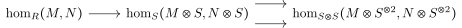where all tensors are over $$R$$. The first map takes a map $$f:M\to N$$ and composes with the map $$N\to N\otimes S$$ from the lemma to get a map $$M\to N\otimes S$$, which automatically extends to a map $$M\otimes S \to N\otimes S$$. Exactness in the middle also comes from the lemma. Alternatively, injectivity of the first map follows from injectivity of $$N\to N\otimes S$$ and left-exactness of $$\hom(M, {\,\cdot\,})$$, as does exactness everywhere else.

A short diversion:

For $$\mathcal{F}\in {\mathrm{QCoh}}(X)$$, we defined $$\mathcal{F}^{\text{ét}} \in {\operatorname{Presh}}(X_{\text{ét}})$$ where $$\mathcal{F}^{\text{ét}}(U\xrightarrow{\pi }X) \mathrel{\vcenter{:}}=\pi^* \mathcal{F}(U)$$ is a sheaf on $$X_{\text{ét}}$$ and $$X_{\mathrm{\operatorname{fppf}}}$$

We want to show that if $$U\to V$$ is an étale cover, we want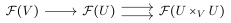to be an equalizer diagram – but this is exactly the previous diagram where $$\mathcal{F}\mathrel{\vcenter{:}}={\mathcal{O}}$$ and $$\mathcal{F}_2 \mathrel{\vcenter{:}}=\mathcal{F}$$.

We have an étale sheaf $${\mathcal{O}}_{X}^{\text{ét}}: (U\to X) \mapsto \Gamma(U, {\mathcal{O}}_U)$$.

### 5.1.2 Proof of Essential Surjectivity

We have $$U\xrightarrow{f} X$$ an fppf cover and descent data $$(\mathcal{F}, \phi)$$ on $$U/X$$ where $$\mathcal{F}$$ is a quasicoherent sheaf on $$U$$ and $$\phi$$ is an isomorphism between its two pullbacks to $$U\times_X U$$ satisfying the cocycle condition on $$U\times_X U \times_X U$$. We want some $$\mathcal{G} \in {\mathrm{QCoh}}(X)$$ such that the pullback admits an isomorphism $$f^* \mathcal{G} \xrightarrow{\sim}\mathcal{F}$$ for which the canonical descent data on the pullback agrees.

We’ll use a similar trick to construct $$\mathcal{G}$$.

Step 1: We’ll reduce to the case of an affine morphism.

Step 2:

Let $$R\xrightarrow{f} S$$ and $$M\in {{S}{\hbox{-}}\operatorname{mod}}$$. We’ll want an isomorphism $$\phi: M\otimes_R S\to S\otimes_R M$$ of $$S\otimes_R S$$ modules satisfying the cocycle condition. We make the following construction: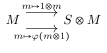Suppose that $$M$$ is of the form $$N\otimes S$$ for $$N\in {R{\hbox{-}}\operatorname{mod}}$$, how would the descent of $$M$$ fit into this diagram and relate to these two maps? Just set $$K$$ to be the equalizer of this diagram, i.e. the subset of $$M$$ that go to the same thing under both maps.

There is obvious map $$K\otimes_R S\to M$$ since $$K \subseteq M$$ and $$M\in {{S}{\hbox{-}}\operatorname{mod}}$$, so you can include $$K\hookrightarrow M$$ and multiply by elements of $$S$$. Moreover, this map is an isomorphism. Given this isomorphism, one obtains compatible descent data on $$M$$.

From the lemma, we have an equalizer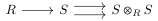to which we can apply $${\,\cdot\,}\otimes_S M$$ to obtain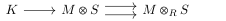where $$K$$ is by definition the above kernel. We want to check that the map $$K\to M$$ appearing here induces an isomorphism $$K\otimes S \to M$$.

This is true if $$R\to S$$ has a section, show this. Given $$U\xrightarrow{f} X$$ with a section $$s$$ and $$\mathcal{F}\in {\mathrm{QCoh}}(U)$$ with descent data, we want $$\mathcal{G}$$ such that $$f^* \mathcal{G} = \mathcal{F}$$. You can take $$\mathcal{G}\mathrel{\vcenter{:}}= s^* \mathcal{F}$$; check that this works.

In general, we want to show $$K\otimes S \to M$$ is an isomorphism, and we want to reduce to the case where we have a section. After applying $${\,\cdot\,}\otimes S$$, $$R\to S$$ acquires a section. Why? We get a map $$S\to S\otimes_R S$$, and there’s a reverse map $$S\otimes_R S\to S$$ given by multiplication. So $$K\otimes S \otimes S \to M\otimes S$$ is an isomorphism, which implies $$K\otimes S\to M$$ is an isomorphism due to the fact that $$S$$ is faithfully flat over $$R$$ which allows us to check exactness before or after tensoring with $$S$$.

The exercises here are among the most important in the course! Totally necessary to do them.

## 5.2 Representable Functors

Suppose $$p:U\to X$$ is an fppf cover. Then the functor $$p^*: {\operatorname{Sch}}/X \to$$ descent data for schemes on $$U/X$$ is fully faithful (but not an equivalence of categories).

### 5.2.1 Proof of Theorem

Reduce to the case where everything is affine, including $$U, X$$, and the scheme over $$X$$. However, it’s enough to reduce to the case of affine schemes over $$X$$, and $$U, X$$ are not necessarily affine.

Step 2: If $$Y,Z$$ are schemes over $$X$$, we want to show the following is an equalizer diagram: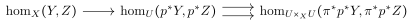Here we’ve suppressed the indices on the $$\pi_i$$ since their images are canonically identified. Injectivity is obvious, using that $$U$$ is surjective, two different morphisms of schemes pulled back along a faithfully flat morphisms are distinct, although we’ll prove this. The real content is that any morphism on $$U$$ that maps to the same thing on $$U\times_X U$$ comes from $$X$$, so we can descend morphisms and the morphisms form a sheaf (which is what we’re trying to prove).

We’ll deduce this from what we proved about quasicoherent sheaves. By the first reduction, we can assume $$Y = \underline{\operatorname{Spec}}_X {\mathcal{O}}_Y$$ is a relative spec, as is $$Z = \underline{\operatorname{Spec}}_X {\mathcal{O}}_Z$$ where the $${\mathcal{O}}$$ here are quasicoherent sheaves of algebras. These are obtained by taking the pushforward of the structure sheaf along $$Y\to X, Z\to X$$. Rewriting the diagram, the homs are now in the category of quasicoherent algebras and we have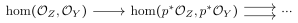where we want this to be an equalizer. This is true because the first map is injective even when ignoring the algebra structure, just looking at a map of quasicoherent sheaves, we know this is injective. If we have an element in the middle that is a morphism of algebras mapping to the same thing, it comes from a quasicoherent sheaf in the first slot. That this is also a map of quasicoherent algebras follows from the fact that descent is functorial. A map of algebras is commuting with a bunch of maps of quasicoherent sheaves, which we know is true on the RHS and is thus true on the LHS since pullback yields an equivalence of categories.

### 5.2.2 Consequences

If $$Z \in {\operatorname{Sch}}/X$$, the $$\hom({\,\cdot\,}, Z)$$ is a sheaf on $$X_{\mathrm{\operatorname{fppf}}}, X_{\text{ét}}, X_{\text{Ét}}$$, etc.

$$p^*$$ is not essentially surjective in general for schemes. Descent data for schemes relative to an étale cover $$U/X$$ is called an algebraic space. Note that some definitions may also required separatedness. When pullback does yield an equivalence of categories, this is referred to as effective descent.

It is essentially surjective for affine schemes and polarized schemes: if you have descent data for a scheme on $$U/X$$ with an ample line bundle (which also has descent data) then you can descend the scheme. Thus projective varieties can be descended, provided you also descend them with an ample line bundle. Replacing $$\operatorname{Spec}$$ with $$\operatorname{Proj}$$ is one way to show this.

• $${\mathbb{G}}_m: U \to {\mathcal{O}}_U(U)^{\times}$$
• $$\mu_\ell: U\to \left\{{f\in {\mathcal{O}}_U(U) {~\mathrel{\Big|}~}f^\ell = 1}\right\}$$.
• $$\underline{\mathbb{Z}/\ell\mathbb{Z}}: U\to \hom_{{\operatorname{Top}}}(U, \mathbb{Z}/\ell\mathbb{Z})$$ sending $$U$$ to continuous maps from $$U$$ to the constant scheme $$\mathbb{Z}/\ell\mathbb{Z}$$, i.e. the $$\ell{\hbox{-}}$$points.
• $$\operatorname{Hilb}^{p(t)}({\mathbb{P}}^n)$$ Hilbert schemes of projective space. We know this is a scheme, so the functor it represents is a sheaf.
• $${\mathbb{P}}^n$$, which represents a line bundle with a surjective map from the trivial bundle of rank $$n+1$$

Work out Galois descent from this point of view.

## 5.3 Étale Cohomology

What missing ingredients do we need to define cohomology for these abelian sheaves?

1. We want to show that the category of abelian sheaves on $$X_{\text{ét}}$$ is abelian.
2. We need enough injectives.

Both of these facts are true for the category of abelian sheaves on any site, i.e. any category with a Grothendieck topology.

The proof of (2) will be relatively easy, but the crucial ingredient for (1) will be the following:

Let $$\tau$$ be a site. Then the forgetful functor $${\operatorname{Sh}}(\tau) \to {\operatorname{Presh}}(\tau)$$ has a left adjoint which we’ll call sheafification.

We’ll just prove this for $$\tau = X_{\text{ét}}$$. The general theorem is much longer!

### 5.3.1 Preliminaries

Some operations we can do with sheaves:

#### 5.3.1.1 Pushforward and pullback

Suppose $$f:\tau_1 \to \tau_2$$ to be a continuous morphism of sites, i.e. a functor $$f^{-1} : \tau_2 \to \tau_1$$ which preserves fiber products (preserving intersections and covering families).

Given $$\mathcal{G}\in {\operatorname{Sh}}(\tau_1)$$, define $$f_* \mathcal{G}\in {\operatorname{Sh}}(\tau_2)$$ by \begin{align*} \qty{f_* \mathcal{G}}(U) \mathrel{\vcenter{:}}=\mathcal{G}( f^{-1}(U)) .\end{align*}

Note that this is the usual formula for pushforwards.

Show that $$f_* \mathcal{G}$$ is a sheaf.

# 6 Lecture 6: Filling in Gaps, Étale Cohomology

Last time a theorem was stated that pullback induced an equivalence of categories $${\mathrm{QCoh}}(X_{{\mathrm{zar}}}) \xrightarrow{\sim} {\mathrm{QCoh}}(X_{\text{ét}}) \xrightarrow{\sim} {\mathrm{QCoh}}(X)(X_{\mathrm{\operatorname{fppf}}})$$; note that these are the little sites. What about the big sites? There are similar equivalences between the three corresponding big sites, but in general, $${\mathrm{QCoh}}(X_{{\mathrm{Zar}}}) \neq {\mathrm{QCoh}}(X_{{\mathrm{zar}}})$$.

For example, a quasicoherent sheaf on the big Zariski site is a quasicoherent sheaf on every $$X{\hbox{-}}$$scheme and morphisms between various pullbacks. This isn’t as affected by what sheaf you have on $$X$$ itself.

Étale descent data for schemes is not quite the same as an algebraic space: it yields an algebraic space, but the data is not literally the same.

## 6.1 Gaps

The category of abelian sheaves on the $$X_{\text{ét}}$$ is an abelian category with enough injectives.

With this in hand, we can use the formalism of derived functors to define étale cohomology:

\begin{align*} H^i(X_\text{ét}, \mathbb{Z}/\ell\mathbb{Z}) \mathrel{\vcenter{:}}= R^i \Gamma(X_\text{ét}, \underline{ \mathbb{Z}/\ell\mathbb{Z}} ) .\end{align*}

The crucial ingredient (mentioned last time) is the following:

For $$\tau$$ a site, the forgetful functor $${\operatorname{Sh}}(\tau) \to {\operatorname{Presh}}(\tau)$$ has a left adjoint (sheafification).

We’ll prove this for the étale site, just Google “sheafification for sites” to find more general proofs. Note that this is actually the inclusion of a full subcategory. Before the proof, we’ll need a few operations in order to imitate the usual proof that sheafification exists for usual sheaves. This is done by constructing the espace étalé out of the stalks and define the sheafification to be sections. The operations we’ll need are:

### 6.1.1 Pushforwards

For $$f: \tau_1 \to \tau_2$$ a continuous map of sites, this was a reversed functor preserving fibers products and covering families. For $$\mathcal{G}\in {\operatorname{Sh}}(\tau_1)$$ we constructed $$f_* \mathcal{G}$$, and the exercise was to show that this is a sheaf.

Let $$f:X\to Y$$ be a map of schemes, this induces a map $$f: X_\text{ét}\to Y_\text{ét}$$ where each $$U/Y$$ comes from $$U\times_Y X$$ over $$X$$.

Suppose $$k=\mkern 1.5mu\overline{\mkern-1.5muk\mkern-1.5mu}\mkern 1.5mu$$ is a field and we have $$\iota_{\mkern 1.5mu\overline{\mkern-1.5muX\mkern-1.5mu}\mkern 1.5mu}:\operatorname{Spec}k\to X$$. We have $${\operatorname{Sh}}\qty{\qty{\operatorname{Spec}k}_{\text{ét}}} = {\operatorname{Set}}$$, since an étale cover of $$\operatorname{Spec}k$$ is a disjoint union of copies of itself. If you show what the value of a sheaf on $$\operatorname{Spec}k$$, you know it on any disjoint union of them since there are a lot of sections. Moreover, any disjoint union of copies of $$\operatorname{Spec}k$$ can be covered by copies $$\operatorname{Spec}k$$ itself by definition.

Show this!

What is the pushforward? \begin{align*} \qty{\iota_{\mkern 1.5mu\overline{\mkern-1.5mux\mkern-1.5mu}\mkern 1.5mu}}_* \mathcal{F}(U\to X) = \mathcal{F}(U\times_X \mkern 1.5mu\overline{\mkern-1.5mux\mkern-1.5mu}\mkern 1.5mu) = F\qty{{\coprod}\operatorname{Spec}k} = \prod \mathcal{F}\operatorname{Spec}k ,\end{align*}

where the number of copies appearing is the number of preimages of $$\mkern 1.5mu\overline{\mkern-1.5mux\mkern-1.5mu}\mkern 1.5mu$$ in $$U$$, and the last equality follows from the sheaf condition.

$$\qty{\iota_{\mkern 1.5mu\overline{\mkern-1.5mux\mkern-1.5mu}\mkern 1.5mu}}_* \mathcal{F}$$ is called the skyscraper sheaf.

### 6.1.2 Pullbacks

In the usual setting, to define a pullback of sheaves you take an direct limit to compute the value on an open set $$U$$, which only yields a presheaf and thus requires sheafifying. We don’t know how to sheafify yet, so we can’t yet define pullbacks in general. We can define pullbacks to a geometric point though:

Let $$\iota_{\mkern 1.5mu\overline{\mkern-1.5mux\mkern-1.5mu}\mkern 1.5mu}: \operatorname{Spec}k \to X$$ with $$k = \mkern 1.5mu\overline{\mkern-1.5muk\mkern-1.5mu}\mkern 1.5mu$$ and set $$\mathcal{F}_{\mkern 1.5mu\overline{\mkern-1.5mux\mkern-1.5mu}\mkern 1.5mu} = \iota_{\mkern 1.5mu\overline{\mkern-1.5mux\mkern-1.5mu}\mkern 1.5mu}^* \mathcal{F}$$ for $$\mathcal{F}\in {\operatorname{Sh}}(X_{\text{ét}})$$. The LHS is a set and the RHS is a sheaf on $$\qty{\operatorname{Spec}k}_{\text{ét}}$$. We then define \begin{align*} \mathcal{F}_{\mkern 1.5mu\overline{\mkern-1.5mux\mkern-1.5mu}\mkern 1.5mu} \def \varinjlim_{(U, u)} \mathcal{F}(U) ,\end{align*} where the limit is taken over diagrams of the form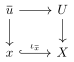where $$\mkern 1.5mu\overline{\mkern-1.5muu\mkern-1.5mu}\mkern 1.5mu$$ is a geometric point and $$Y\to X$$ is étale. $$\mathcal{F}_{\mkern 1.5mu\overline{\mkern-1.5mux\mkern-1.5mu}\mkern 1.5mu}$$ is referred to as the stalk of $$\mathcal{F}$$ at $$\mkern 1.5mu\overline{\mkern-1.5mux\mkern-1.5mu}\mkern 1.5mu$$.

We don’t have to work at a closed point. Taking $$\operatorname{Spec}k$$ to be the algebraic closure of the function field of $$X$$ is $$X$$ is irreducible.

Let $$\mathcal{F} = \underline{\mathbb{Z}/\ell\mathbb{Z}}$$ and $$\mkern 1.5mu\overline{\mkern-1.5mux\mkern-1.5mu}\mkern 1.5mu\hookrightarrow X$$ any geometric point. Then the pullback is given by $$\iota_{\mkern 1.5mu\overline{\mkern-1.5mux\mkern-1.5mu}\mkern 1.5mu}^* \qty{\underline{\mathbb{Z}/\ell\mathbb{Z}}} = \mathbb{Z}/\ell\mathbb{Z}$$. If $$U$$ had more than one connected component, then the first definition would involve a limit over $$\mathcal{F}(U)$$ which are all copies of $$\mathbb{Z}/\ell\mathbb{Z}$$. But given this, you can always find a connected covering. So the $$(U, \mkern 1.5mu\overline{\mkern-1.5muu\mkern-1.5mu}\mkern 1.5mu)$$ which are connected are actually cofinal.18

Let $$\mathcal{F} = {\mathcal{O}}_{X}^{\text{ét}}$$, then the pullback is $$\iota_{\mkern 1.5mu\overline{\mkern-1.5mux\mkern-1.5mu}\mkern 1.5mu}^* {\mathcal{O}}_X^{\text{ét}} = {\mathcal{O}}_{X\mkern 1.5mu\overline{\mkern-1.5mux\mkern-1.5mu}\mkern 1.5mu}^{\mathrm{sh}}$$, which is the strict Henselization (where we’re picking up the version that has an algebraically closed residue field).

Some useful things about stalks: we can check things like isomorphisms locally on them.

Suppose $$\mathcal{F}, \mathcal{G}$$ are sheaves of abelian groups on $$X_{\text{ét}}$$. Then TFAE

1. $$\mathcal{F}\to \mathcal{G}$$ is an epimorphism,
2. $$\mathcal{F}\to \mathcal{G}$$ is locally surjective, i.e. given a section $$s\in \mathcal{G}(U)$$ there exists $$U'\to U$$ such that $${ \left.{{s}} \right|_{{U'}} }$$ is the image of some $$s' \in \mathcal{F}(U)$$.19
3. $$\mathcal{F}_{\mkern 1.5mu\overline{\mkern-1.5mux\mkern-1.5mu}\mkern 1.5mu} \to \mathcal{G}_{\mkern 1.5mu\overline{\mkern-1.5mux\mkern-1.5mu}\mkern 1.5mu}$$ is surjective for all geometric points $$\mkern 1.5mu\overline{\mkern-1.5mux\mkern-1.5mu}\mkern 1.5mu\to X$$.

Suppose we have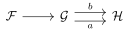where the 2 compositions agree, then we want to show that $$a=b$$. Let $$s$$ be a section of $$\mathcal{G}$$ on $$U$$, we want to know that $$a(s) = b(s)$$. By (2), we can replace $$s$$ with $$s'$$ coming from $$\mathcal{F}$$, so $$a(s') = b(s')$$ since the compositions agree.

We want to show that given an epimorphisms, the map on every stalk is surjective. Assume $$\mathcal{F}_{\mkern 1.5mu\overline{\mkern-1.5mux\mkern-1.5mu}\mkern 1.5mu} \to \mathcal{G}_{\mkern 1.5mu\overline{\mkern-1.5mux\mkern-1.5mu}\mkern 1.5mu}$$ is not surjective, and thus has a nontrivial cokernel $$\Lambda$$. We can construct 2 maps to the skyscraper sheaf: